# AVERAGE

The AVERAGE function in Excel calculates the average (arithmetic mean) of a group of numbers. The AVERAGE function ignores logical values, empty cells and cells that contain text.

### Average

Instead of using the AVERAGE function, use SUM and COUNT.

1. For example, the AVERAGE function below calculates the average of the numbers in cells A1 through A3.2. The formula below produces the exact same result.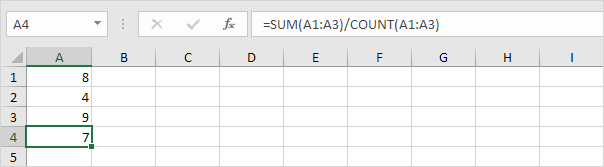3. The following AVERAGE function calculates the average of the numbers in cells A1 through A3 and the number 8.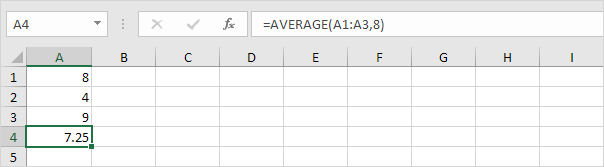4. The AVERAGE function ignores logical values (TRUE or FALSE), empty cells and cells that contain text.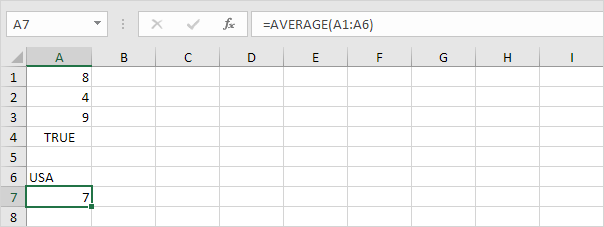### AverageA

The AVERAGEA function also returns the average (arithmetic mean) of a group of numbers. However, the logical value FALSE and cells that contain text evaluate to 0 and the logical value TRUE evaluates to 1. The AVERAGEA function also ignores empty cells.

1. For example, take a look at the AVERAGEA function below.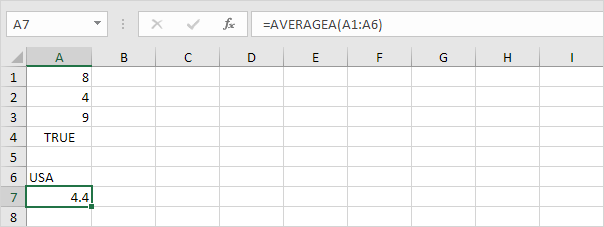2. You can use the normal AVERAGE function to check this result.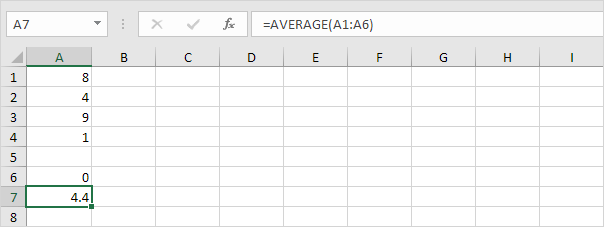### Average Top 3

Use AVERAGE and LARGE in Excel to calculate the average of the top 3 numbers in a data set.

1. First, the AVERAGE function below calculates the average of the numbers in cells A1 through A6.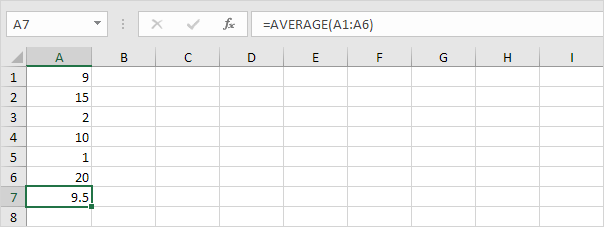2. For example, to find the third largest number, use the following LARGE function.3. The formula below calculates the average of the top 3 numbers.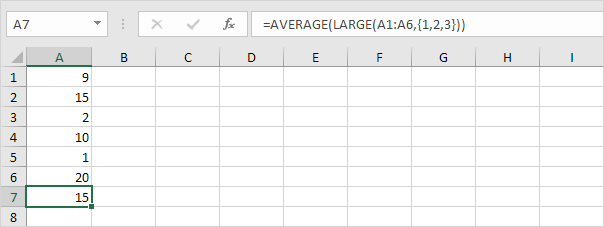Explanation: the LARGE function returns the array constant {20,15,10}. This array constant is used as an argument for the AVERAGE function, giving a result of 15.

### AverageIf

To calculate the average of cells that meet one criteria, use the AVERAGEIF function in Excel.### Weighted Average

To calculate a weighted average in Excel, use SUMPRODUCT and SUM.### Moving Average

Use the Analysis Toolpak to calculate the moving average of a time series in Excel.Note: a moving average is used to smooth out irregularities (peaks and valleys) to easily recognize trends.

Go to Next Chapter: Round